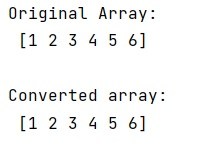# How to convert a numpy.ndarray to string(or bytes) and convert it back to numpy.ndarray?

Learn, how to convert a numpy.ndarray to string(or bytes) and convert it back to numpy.ndarray in Python?
Submitted by Pranit Sharma, on February 25, 2023

NumPy is an abbreviated form of Numerical Python. It is used for different types of scientific operations in python. Numpy is a vast library in python which is used for almost every kind of scientific or mathematical operation. It is itself an array which is a collection of various methods and functions for processing the arrays.

## Converting a numpy.ndarray to string(or bytes) and convert it back to numpy.ndarray

The string is a group of characters, these characters may consist of all the lower case, upper case, and special characters present on the keyboard of a computer system. A string is a data type and the number of characters in a string is known as the length of the string.

We can convert a numpy ndarray using tostring() method and to convert this string back into the form of a numpy ndarray, we can use "numpy.fromstring() method and also we need to define the data type of the elements.

Let us understand with the help of an example,

## Python code to convert a numpy.ndarray to string(or bytes) and convert it back to numpy.ndarray

```# Import numpy
import numpy as np

# Creating a numpy array
arr = np.array([1, 2, 3, 4, 5, 6])

# Display original array
print("Original Array:\n",arr,"\n")

# Converting into string
str = arr.tostring()

# Converting string into array
arr = np.fromstring(str,dtype=int)

# Display converted array
print("Converted array:\n",arr,"\n")
```

Output:Languages: » C » C++ » C++ STL » Java » Data Structure » C#.Net » Android » Kotlin » SQL
Web Technologies: » PHP » Python » JavaScript » CSS » Ajax » Node.js » Web programming/HTML
Solved programs: » C » C++ » DS » Java » C#
Aptitude que. & ans.: » C » C++ » Java » DBMS
Interview que. & ans.: » C » Embedded C » Java » SEO » HR
CS Subjects: » CS Basics » O.S. » Networks » DBMS » Embedded Systems » Cloud Computing
» Machine learning » CS Organizations » Linux » DOS
More: » Articles » Puzzles » News/Updates